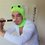# Integral help?

I was doing a problem in which i used a bit unconventional approach, but in my process, i came across the following integral. but i am not really good in integration, as i am new to the subject, so i want to ask, if the integral is derivable? and if so, how to do this:

$\large{\int\dfrac{sin(\frac{nx}{2}).cos\frac{(n+1)x}{2}}{sin(\frac{x}{2})}.dx}$Note by Aritra Jana
6 years, 3 months ago

This discussion board is a place to discuss our Daily Challenges and the math and science related to those challenges. Explanations are more than just a solution — they should explain the steps and thinking strategies that you used to obtain the solution. Comments should further the discussion of math and science.

When posting on Brilliant:

• Use the emojis to react to an explanation, whether you're congratulating a job well done , or just really confused .
• Ask specific questions about the challenge or the steps in somebody's explanation. Well-posed questions can add a lot to the discussion, but posting "I don't understand!" doesn't help anyone.
• Try to contribute something new to the discussion, whether it is an extension, generalization or other idea related to the challenge.

MarkdownAppears as
*italics* or _italics_ italics
**bold** or __bold__ bold
- bulleted- list
• bulleted
• list
1. numbered2. list
1. numbered
2. list
Note: you must add a full line of space before and after lists for them to show up correctly
paragraph 1paragraph 2

paragraph 1

paragraph 2

[example link](https://brilliant.org)example link
> This is a quote
This is a quote
    # I indented these lines
# 4 spaces, and now they show
# up as a code block.

print "hello world"
# I indented these lines
# 4 spaces, and now they show
# up as a code block.

print "hello world"
MathAppears as
Remember to wrap math in $$ ... $$ or $ ... $ to ensure proper formatting.
2 \times 3 $2 \times 3$
2^{34} $2^{34}$
a_{i-1} $a_{i-1}$
\frac{2}{3} $\frac{2}{3}$
\sqrt{2} $\sqrt{2}$
\sum_{i=1}^3 $\sum_{i=1}^3$
\sin \theta $\sin \theta$
\boxed{123} $\boxed{123}$

Sort by:

Can you post the original problem??

- 6 years, 3 months ago

the original problem asked for the sum of the series :

$\large{\sum\limits_{r=1}^{n}\frac{1}{r}sin(r\frac{\pi}{3})}$

I was thinking if i could use the identity :

$\large{\sum\limits_{r=1}^{n}cos(rx)=\dfrac{sin(\frac{nx}{2}).cos\frac{(n+1)x}{2}}{sin(\frac{x}{2})}}$

and then integrate this to get the above sum, but as you can see, this was not fruitful.

i shall post this original problem in a new thread. :D

- 6 years, 3 months ago

$\dfrac{\pi}{3} = x$

$S = \displaystyle \sum_{r=1}^{n}\dfrac{sin(rx)}{r}$

$C = \displaystyle \sum_{r=1}^{n}\dfrac{cos(rx)}{r}$

$C + iS = \displaystyle \sum_{r=1}^{n} \dfrac{e^{irx}}{r}$

$Now~ \displaystyle \sum_{r=1}^{n} x^{r - 1} = \dfrac{1 - x^n}{1 - x}$

$\displaystyle \sum_{r=1}^{n} \dfrac{x^r}{r} = \int \dfrac{1 - x^n}{1 - x}$

Then keeping $e^{ix} = x$ and comparing the imaginary part we can get the answer but I am unable to find this - $\int \dfrac{x^n}{1 - x}$

- 6 years, 3 months ago

@U Z Any ideas @Jake Lai

- 6 years, 3 months ago

There was a problem related to this sum.

- 6 years, 3 months ago

really? i wasn't aware of that this actually came in our FIITJEE open test Mains

- 6 years, 3 months ago